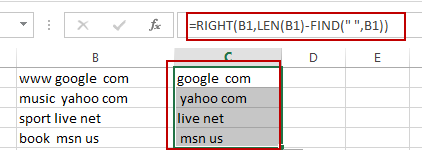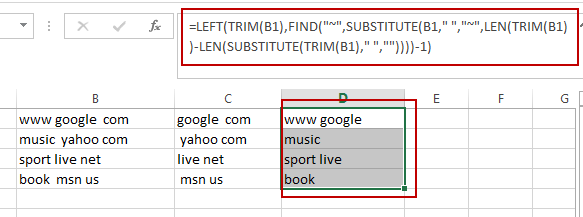# How to Remove the First/Last Word from Text string in Cell

This post will guide you how to remove the first and the last word from a text string in cells in Excel. How do I use a formula to remove first and last word of a text string in Excel.

## Remove the First Word from Text String

If you want to remove the first word from a text string in cells in Excel, you can use a formula based on the RIGHT function, the LEN function and the FIND function. Like this:

`=RIGHT(B1,LEN(B1)-FIND(“ ”,B1))`

Type this formula into a blank cell and press Enter key on your keyboard and then drag the AutoFill Handle down to other cells to apply this formula.## Remove the Last Word from Text String

If you want to remove the last word from a text string, you can use a formula based on the LEFT function, the TRIM function, the FIND function, and the SUBSTITUTE function. Like this:

`=LEFT(TRIM(B1),FIND("~",SUBSTITUTE(B1," ","~",LEN(TRIM(B1))-LEN(SUBSTITUTE(TRIM(B1)," ",""))))-1)`

Type this formula into a blank cell and press Enter key on your keyboard and then drag the AutoFill Handle down to other cells to apply this formula.### Related Functions

• Excel LEFT function
The Excel LEFT function returns a substring (a specified number of the characters) from a text string, starting from the leftmost character.The LEFT function is a build-in function in Microsoft Excel and it is categorized as a Text Function.The syntax of the LEFT function is as below:= LEFT(text,[num_chars])…t)…
• Excel FIND function
The Excel FIND function returns the position of the first text string (sub string) within another text string.The syntax of the FIND function is as below:= FIND(find_text, within_text,[start_num])…
• Excel RIGHT function
The Excel RIGHT function returns a substring (a specified number of the characters) from a text string, starting from the rightmost character.The syntax of the RIGHT function is as below:= RIGHT (text,[num_chars])…
• Excel Substitute function
The Excel SUBSTITUTE function replaces a new text string for an old text string in a text string.The syntax of the SUBSTITUTE function is as below:= SUBSTITUTE  (text, old_text, new_text,[instance_num])….
• Excel LEN function
The Excel LEN function returns the length of a text string (the number of characters in a text string).The syntax of the LEN function is as below:= LEN(text)…
• Excel TRIM function
The Excel TRIM function removes all spaces from text string except for single spaces between words.  You can use the TRIM function to remove extra spaces between words in a string.The syntax of the TRIM function is as below:= TRIM (text)….

Sidebar